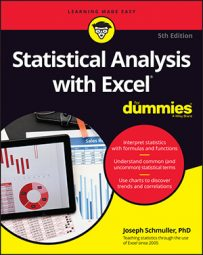##### Statistical Analysis with Excel For DummiesExcel knows how to help when you have more than two samples. FarKlempt Robotics, Inc., surveys its employees about their level of satisfaction with their jobs. They ask developers, managers, maintenance workers, and tech writers to rate job satisfaction on a scale of 1 (least satisfied) to 100 (most satisfied).

Six employees are in each category. The image below shows a spreadsheet with the data in columns A through D, rows 1–7. The null hypothesis is that the samples all come from the same population. The alternative hypothesis is that they do not.

The appropriate non-parametric test is the Kruskal-Wallis One-Way Analysis of Variance. Start by ranking all 24 scores in ascending order. Again, if the null hypothesis true, the ranks should be distributed about equally throughout the groups.

The formula for this statistic is

N is the total number of scores, and n is the number of scores in each group. To keep things easy, you specify the same number of scores in each group, but that’s not necessary for this test. R is the sum of the ranks in a group. H is distributed approximately as chi-square with df = number of groups — 1, when each n is greater than 5.

Looking back at the image, the ranks for the data are in rows 9–15 of columns A through D. Row 16 holds the sums of the ranks in each group. Define N_Total as the name for the value in cell F2, the total number of scores. Define n_group as the name for the value in G2, the number of scores in each group.

To calculate H, type

`=(12/(N_Total*(N_Total+1)))*(SUMSQ(A16:D16)/n_group)-3*(N_Total+1)`

into cell G6.

For the hypothesis test, type

`=CHISQ.DIST.RT(G6,3)`

into G7. The result is less than .05, so you reject the null hypothesis.